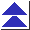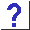# 20. Miscellaneous

20.1 How can I return multiple values from a function?

20.2 What's a good data structure to use for storing lines of text? I started to use fixed-size arrays of arrays of char, but they're just too restrictive.

20.3 How can I open files mentioned on the command line, and parse option flags?

20.4 What's the right way to use errno?

20.5 How can I write data files which can be read on other machines with different word size, byte order, or floating point formats?

20.6 If I have a char * variable pointing to the name of a function, how can I call that function? Code like

```	extern int func(int, int);
char *funcname = "func";
int r = (*funcname)(1, 2);
```
or
```	r = (*(int (*)(int, int))funcname)(1, 2);
```
doesn't seem to work.

20.6b How can I ensure that integer arithmetic doesn't overflow?

20.7 How can I manipulate individual bits?

20.8 How can I implement sets or arrays of bits?

20.9 How can I determine whether a machine's byte order is big-endian or little-endian?

20.9b How do I swap bytes?

20.10 How can I convert integers to binary or hexadecimal?

20.11 Can I use base-2 constants (something like 0b101010)?
Is there a printf format for binary?

20.12 What is the most efficient way to count the number of bits which are set in an integer?

20.13 What's the best way of making my program efficient?

20.14 Are pointers really faster than arrays? How much do function calls slow things down? Is ++i faster than i = i + 1?

20.15 I've been replacing multiplications and divisions with shift operators, because shifting is more efficient.

20.15b People claim that optimizing compilers are good and that we no longer have to write things in assembler for speed, but my compiler can't even replace i/=2 with a shift.

20.15c How can I swap two values without using a temporary?

20.16 Which is more efficient, a switch statement or an if/else chain?

20.17 Is there a way to switch on strings?

20.18 Is there a way to have non-constant case labels (i.e. ranges or arbitrary expressions)?

20.19 Are the outer parentheses in return statements really optional?

20.20 Why don't C comments nest? How am I supposed to comment out code containing comments? Are comments legal inside quoted strings?

20.20b Why isn't there a numbered, multi-level break statement to break out of several loops at once? What am I supposed to use instead, a goto?

20.21 There seem to be a few missing operators, like ^^, &&=, and ->=.

20.21a Does C have circular shift operators?

20.21b Is C a great language, or what? Where else could you write something like a+++++b ?

20.22 If the assignment operator were :=, wouldn't it then be harder to accidentally write things like if(a = b) ?

20.23 Does C have an equivalent to Pascal's with statement?

20.24 Why doesn't C have nested functions?

20.24b What is assert() and when would I use it?

20.25 How can I call FORTRAN (C++, BASIC, Pascal, Ada, LISP) functions from C? (And vice versa?)

20.26 Does anyone know of a program for converting Pascal or FORTRAN (or LISP, Ada, awk, ``Old'' C, ...) to C?

20.27 Is C++ a superset of C? What are the differences between C and C++? Can I use a C++ compiler to compile C code?

20.28 I need a sort of an ``approximate'' strcmp routine, for comparing two strings for close, but not necessarily exact, equality.

20.29 What is hashing?

20.30 How can I generate random numbers with a normal or Gaussian distribution?

20.31 How can I find the day of the week given the date?

20.32 Is (year % 4 == 0) an accurate test for leap years? (Was 2000 a leap year?)

20.33 Why can tm_sec in the tm structure range from 0 to 61, suggesting that there can be 62 seconds in a minute?

20.34 Here's a good puzzle: how do you write a program which produces its own source code as output?

20.35 What is ``Duff's Device''?

20.36 When will the next International Obfuscated C Code Contest (IOCCC) be held? How do I submit contest entries? Who won this year's IOCCC? How can I get a copy of the current and previous winning entries?

20.37 What was the entry keyword mentioned in K&R1?

20.38 Where does the name ``C'' come from, anyway?

20.39 How do you pronounce ``char''? What's that funny name for the ``#'' character?

20.39b What do ``lvalue'' and ``rvalue'' mean?

20.40 Where can I get extra copies of this list?